#Orthogonal TrajectoriesWe have seen before (see separable equations for example) that the solutions of a differential equation may be given by an implicit equation with a parameter something like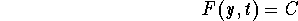This is an equation describing a family of curves. Whenever we fix the parameter C we get one curve and vice-versa. For example, consider the families of curves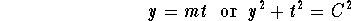where m and C are parameters. Clearly, we may change the names of the variables and still have the same geometric curves. For example, the above families define the same geometric object as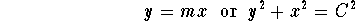Note that the first family describes all the lines passing by the origin (0,0) while the second the family describes all the circles centered at the origin (including the limit case when the radius 0 which reduces to the single point (0,0)) (see the pictures below).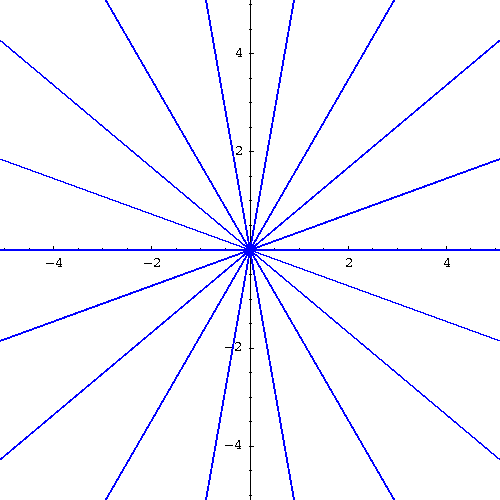and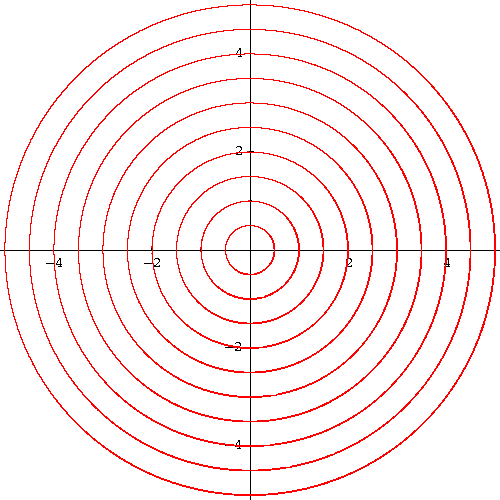In this page, we will only use the variables x and y. Any family of curves will be written as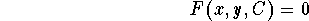One may ask whether any family of curves may be generated from a differential equation? In general, the answer is no. Let us see how to proceed if the answer were to be yes. First differentiate with respect to x, and get a new equation involving in general x, y,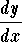, and C. Using the original equation, we may able to eliminate the parameter C from the new equation.

Example. Find the differential equation satisfied by the family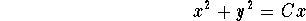Answer. We differentiate with respect to x, to get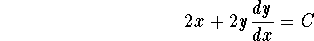Since we have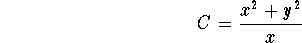then we get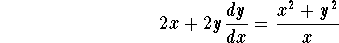You may want to do some algebra to make the new equation easy to read. The next step is to rewrite this equation in the explicit form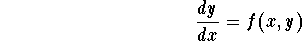this is the desired differential equation.

Example. Find the differential equation (in the explicit form) satisfied by the familyAnswer. We have already found the differential equation in the implicit formAlgebraic manipulations give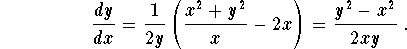Let us reconsider the example of the two familiesIf we draw the two families together on the same graph we get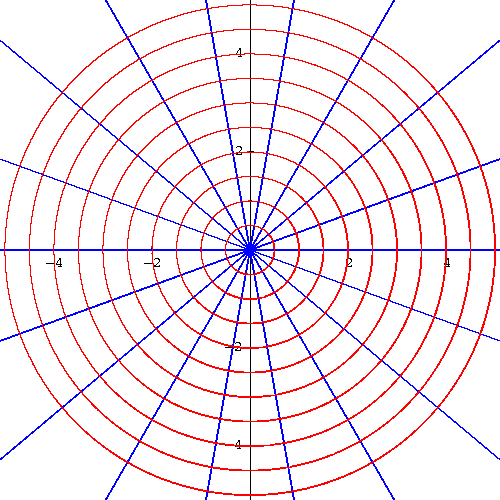As we see here something amazing happened. Indeed, it is clear that whenever one line intersects one circle, the tangent line to the circle (at the point of intersection) and the line are perpendicular or orthogonal. We say the two curves are orthogonal at the point of intersection.

Definition. Consider two families of curves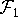and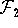. We say thatandare orthogonal whenever any curve fromintersects any curve from, the two curves are orthogonal at the point of intersection.

For example, we have seen that the families y = m x and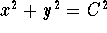are orthogonal. One may then ask the following natural question:

Given a family of curves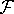, is it possible to find a family of curves which is orthogonal to?

The answer to this question has many implications in many areas such as physics, fluid-dynamics, etc... In general this question is very difficult. But in some cases, we may be able to carry on the calculations and find the orthogonal family. Let us show how.

Consider the family of curves. We assume that an associated differential equation may be found, sayWe know that for any curve from the family passing by the point (x,y), the slope of the tangent at this point is f(x,y). Hence the slope of the line perpendicular (or orthogonal) to this tangent is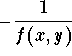which happens to be the slope of the tangent line to the orthogonal curve passing by the point (x,y). In other words, the family of orthogonal curves are solutions to the differential equation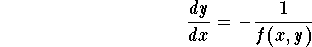From this we see what we have to do. Indeed consider a family of curves. In order to find the orthogonal family, we use the following practical steps

Step 1. Find the associated differential equation.
Step 2. Rewrite this differential equation in the explicit formStep 3. Write down the differential equation associated to the orthogonal familyStep 4. Solve the new equation. The solutions are exactly the family of orthogonal curves.
Step 5. You may be asked to give a geometric view of the two families. Also you may be asked to find a specific curve from the orthogonal family (something like an IVP).

Example. Find the orthogonal family to the family of circles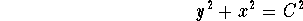Answer. First, we look for the differential equation satisfied by the circles. We differentiate with respect to the variable x to get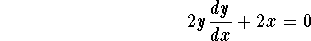We rewrite this equation in the explicit form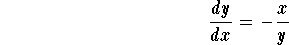Next we write down the equation for the orthogonal family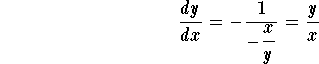This is a linear as well as a separable equation. If we use the technique of linear equations, we get the integrating factor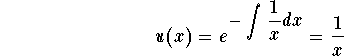which gives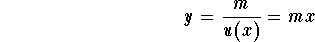We recognize the family of lines and we confirm our earlier observation (that the two families are indeed orthogonal).

This example is somehow easy and was given here to illustrate the technique.

Example. Find the orthogonal family to the family of circles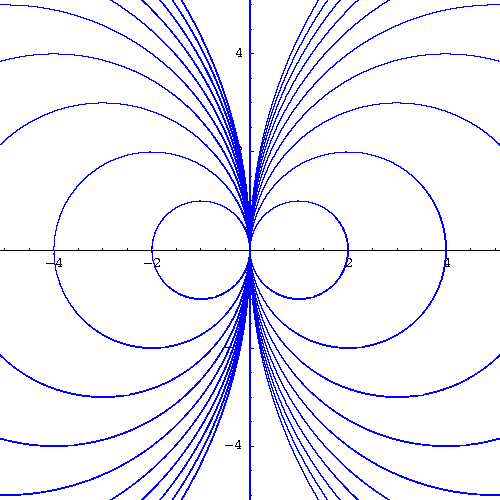Answer. We have seen before that the explicit differential equation associated to the family of circles is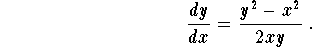Hence the differential equation for the orthogonal family is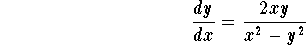We recognize an homogeneous equation. Let us use the technique developed to solve this kind of equations. Consider the new variable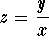(or equivalently y = x z). Then we have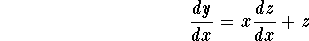and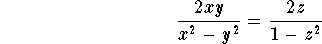Hence we have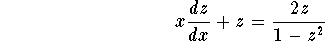Algebraic manipulations imply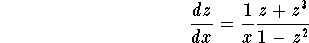This is a separable equation. The constant solutions are given by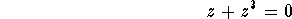which gives z=0. The non-constant solutions are found once we separate the variables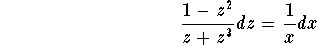and then we integrate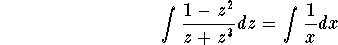Before we perform the integration for the left-hand side, we need to use partial decomposition technique. We have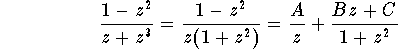We will leave the details to you to show that A = 1, B=-2, and C=0. Hence we have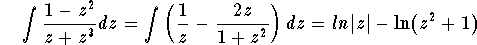Hence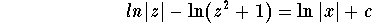which is equivalent to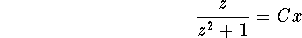where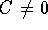. Putting all the solutions together we get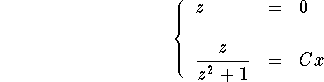Going back to the variable y, we get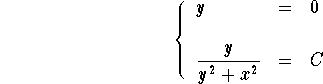which is equivalent to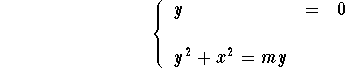We recognize a family of circles centered on the y-axis and the line y=0 (the x-axis which was easy to guess, isn't it?)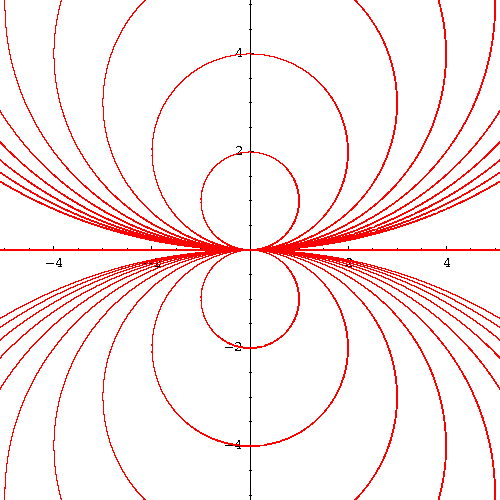If we put both families together, we appreciate better the orthogonality of the curves (see the picture below).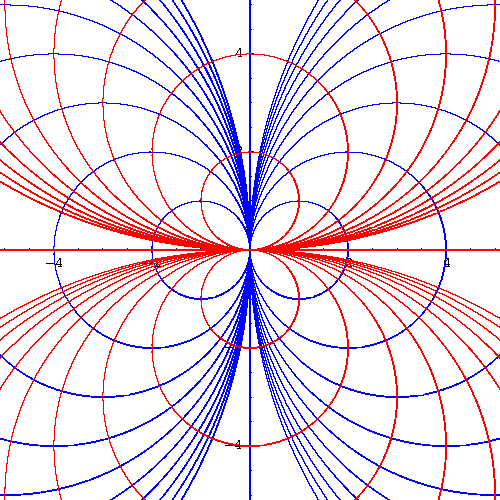[Differential Equations] [First Order D.E.]
[Geometry] [Algebra] [Trigonometry ]
[Calculus] [Complex Variables] [Matrix Algebra]S.O.S MATHematics home page

Do you need more help? Please post your question on our S.O.S. Mathematics CyberBoard.Author: Mohamed Amine Khamsi